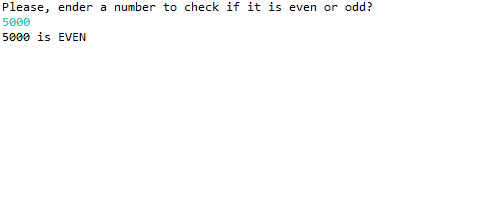# Remainder Operator in Java

Sarwan Soomro Jan 30, 2023 Feb 27, 2022

This article will introduce the remainder operator in Java and its uses.

## Compute Remainder of Integer Division Using the Remainder Operator in Java

In Java, modulo or the remainder operator is given by the symbol `%`. It calculates the remainder of a number divided by another.

Suppose, we have two values: `value1 = 20`, `value 2 = 35`. Here, `value1` is the dividend and `value2` is the divisor.

Example:

``````//load package
package JavaPercentagesymbol;
import java.util.Scanner;
//main class starts here
public class SymbolExample {
//main function
public static void main(String[] args){
//value1/value2%remainder
int value1,value2,remainder;
value1=20;
value2=35;
System.out.println("value1=20; value2=35");
remainder=value1%value2;
System.out.println("The remainder is: "+remainder);
}
//primary function ends here
}
//main class ends here
``````

Output:

``````value1=20; value2=35
The remainder is: 20
``````

## Get String Length Using Reminder Operator in Java

In the following code block, the `String[] username` length is 4, and the size of the `int length` varies. However, the modulo operator correctly determines the actual length.

Since the length of the username is four, the remainder operator divides it with the length of the string instead of the length of a varying integer length.

Example:

``````package JavaPercentagesymbol;
public class SymbolExample {
public static void main (String[] args) {
String[] username = { "John" , "Smith" , "Martin" ,"Herry"};
int length;
System.out.println("modulo 1: " + 6 % 4);
System.out.println(" 6 % 4 is " + length + ": " +username[length]);
System.out.println("modulo 2: " + 30 % 4);
System.out.println(" 70 % 4 is " + length + ": " +username[length]);
System.out.println("modulo 3: " + 40 % 4);
System.out.println("60 % 4 is " + length + ": " +username[length]);
System.out.println("modulo 4: " + 49 % 4);
System.out.println("60 % 4 is " + length + ": " +username[length]);
}
}
``````

Output:

``````modulo 1: 2
6 % 4 is 2: Martin
modulo 2: 2
70 % 4 is 3: Herry
modulo 3: 0
60 % 4 is 0: John
modulo 4: 1
60 % 4 is 1: Smith
``````

The modulo `%` determined the actual length of `String[]username` out of the `username.length` array indexes.

But as you see, the length of the `int length` is 7, 40, and 49.

That is how the program intelligently returns the remainder of the `username.length` rather than giving us the rest of unordered `int length` values.

## Check Even/Odd Number Using Remainder Operator in Java

The program below is an entry-level Java logic builder. It determines whether the given input in (integers) is even or odd.

Example:

``````package JavaPercentagesymbol;
import java.util.Scanner;
public class SymbolExample {
public static void main(String []args ) {
int value;
try (Scanner in = new Scanner(System.in)) {
System.out.println("Please, ender a number to check if it is even or odd?");
value=in.nextInt();
}
// determine the even value%2==0 will always be even
if((value % 2)==0){
System.out.println(+value+" is EVEN ");
//else, it is definitely going to be odd
}else{
System.out.println(+value+" is ODD");
}
}
}
``````

Output:The Scanner generates a new Scanner object using the input stream passed as an argument in the code above.

And the `nextInt()` method takes a string of digits and changes it to an `int` data type. It gets the same passed argument from the Scanner.

Sarwan Soomro is a freelance software engineer and an expert technical writer who loves writing and coding. He has 5 years of web development and 3 years of professional writing experience, and an MSs in computer science. In addition, he has numerous professional qualifications in the cloud, database, desktop, and online technologies. And has developed multi-technology programming guides for beginners and published many tech articles.